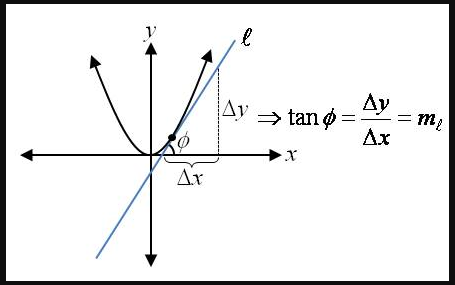# The angle of inclination ϕ which makes the angle with the positive direction x-axis.### Single Variable Calculus: Concepts...

4th Edition
James Stewart
Publisher: Cengage Learning
ISBN: 9781337687805### Single Variable Calculus: Concepts...

4th Edition
James Stewart
Publisher: Cengage Learning
ISBN: 9781337687805

#### Solutions

Chapter 2.7, Problem 55E
To determine

## To calculate:The angle of inclination ϕ which makes the angle with the positive direction x-axis.

Expert Solution

The angle of inclination ϕ is 63 .

### Explanation of Solution

Given: l Be any tangent line of a parabola y=x2 at the point (1,1) .

Let l be any tangent line of a parabola y=x2 at the point (1,1) .

It has to find angle of inclination ϕ which makes the angle with the positive direction

x-axis.The tangent (as a trigonometric function) of the angle of inclination ϕ of a tangent line l

(i.e.tanϕ) is the slope of l (see diagram on the right).

Here, the l is the tangent line to y=x2 at x=1 ,

Therefore, its slope is y'|x=1=2x|x=1=2(1)=2

Therefore, tanϕ=2ϕ=tan1263

Hence, the angle of inclination ϕ is 63 .

### Have a homework question?

Subscribe to bartleby learn! Ask subject matter experts 30 homework questions each month. Plus, you’ll have access to millions of step-by-step textbook answers!